American Journal of Theoretical and Applied Statistics
Volume 5, Issue 5, September 2016, Pages: 285-289

Towards Efficiency in the Residual and Parametric Bootstrap Techniques

Acha Chigozie K.*, Omekara Chukwuemeka O.

Department of Statistics, Michael Okpara University of Agriculture Umudike, Umudike, Nigeria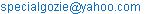(Acha C. K.)

*Corresponding author

Acha Chigozie K., Omekara Chukwuemeka O. Towards Efficiency in the Residual and Parametric Bootstrap Techniques. American Journal of Theoretical and Applied Statistics. Vol. 5, No. 5, 2016, pp. 285-289. doi: 10.11648/j.ajtas.20160505.16

Received: July 20, 2016; Accepted: July 30, 2016; Published: August 17, 2016

Abstract: There are many bootstrap methods that can be used for statistical analysis especially in econometrics, biometrics, Statistics, Sampling and so on. The sole aim of this paper is to ascertain the accuracy and efficiency of the estimates from the independent and identically distributed (iid) simple linear regression (SLR) model under a variety of assessment conditions using bootstrap techniques. Analysis was carried out using S-plus statistical package on hypothetical data sets from a normal distribution with different group proficiency levels to buttress the arguments in the paper. In the course of the analysis, 268,800 scenarios were replicated 1000 times. The result shows a significant difference between the performances of the bootstrap methods used, namely; residual and parametric bootstrap techniques. From the analysis, the largest bias and standard error were always associated with model HP311 while the smallest bias and standard error values were associated with models HR311. The exception was found in the group proficiency level 3 - N (1, 0.25), when the sample sizes were 200, 1000 and 10000 instead of model HR311 producing the smallest bias and standard error, model RP311 did. The significantly better performance of the residual bootstrap indicates the possible use of this technique in assessment of comparative performance and the capability of yielding very accurate, consistent, faster and extra-ordinarily reliable statistical inference under several assessment conditions.

Keywords: Residual Bootstrap, Efficiency, Sufficiency, Parametric Bootstrap, Technique

Contents

1. Introduction

Bootstrap is not simply another statistical technique but is rather a general approach to statistical inference with very broad applicability and very mild modeling assumptions. It is the result from the way the sample information is processed. For instance, in the case of samples from a normal distribution, all the information about the distribution of the sample mean is summarized in the sample mean and variance (standard error for samples), which are jointly sufficient statistics. Thus, other ways of processing sample information in this case does not yield any better results. In most econometric applications, where there is no readily available finite sample distribution of the test statistics that’s when one gets the most mileage out of the bootstrap methods. Even though, it is computationally more demanding than other sampling techniques. There are several forms of the bootstrap techniques but two methods (residual and parametric) are usually used when the dataset is independent and identically distributed (iid). This study examines them to ascertain which one is more efficient and sufficient statistic with respect to their proficiency level, bias and standard error on SLR models. Secondly, to estimate the test statistics of the functional models and to determine the best model under those conditions.

This will be aided by an S-plus program (stat 4); the residual and parametric methods and functions were also incorporated. In addition, a hypothetical data sets from a normal distribution with different group proficiency levels will be used to buttress the arguments in the paper.

2. Literature Review

 considered as a special case where a parametric regression model yi= mL (xi) + ei, i = 1, where mL (xi) is the regression function and ei denotes the associated ith error. For fixed design and parametric regression function, we may proceed by resampling the residuals eri = yi -mLh (xi) where Lh is a parameter estimator. Naive bootstrap samples e*i drawn from the empirical distribution of the centered residuals eri, are used to get the bootstrap regression model y*i = mLh (xi)+ e*i. This approach is called model-based parametric bootstrapping. Each of the bootstrap samples can provide an estimate of the regression parameter (s) following the same estimation procedure that was used with the original fitted model (e.g., ordinary least squares). From all the bootstrap replicates we get a simulation approximation to the bootstrap distribution of the regression parameter (s) and this is then used to make inferences about the parameter (s) based on this approximation to the sampling distribution (s) for the parameter estimate (s). When an explicit parametric equation is not available, an alternative is the block bootstrap, which consists of resampling blocks of subsamples, trying to capture the dependence in the data. When bootstrap replicates obtained from block bootstrap is not stationary, a bootstrap method called stationary bootstrap proposed by  will be used. , introduced the tapered block bootstrap. The idea of the block bootstrap has also been extended to the spatial setting, . In model-based inference,  underscored the importance of the choice of residuals for the residual bootstrapping. Different modifications of this simple idea allow for adapting to random design, heteroscedastic models or situations where the regression function is not totally specified or is unknown , , . For example, similar to the ideas of bootstrap in regression models, given an explicit dependence structure such an autoregressive model, [yi = m (yi-1, yi-2,..., yi-p)] and proceed by resampling from the residuals. Moreover, an overview of the residual bootstrap methods for estimation and prediction in time series and regression can be found in , , , , . Though,  and , ,  worked on the general efficiency of the bootstrap. In this study, the particular bootstrap method that is more efficient over the other in independent and identical distribution (iid) on the SLR will be established and added to literature.

3. Methodology

In this section, hypothetical data sets will be bootstrapped using the procedures below to develop residual and parametric bootstrap (PB) models aided by an S-plus package.

3.1. The Residual Bootstrap

Assuming the error terms in SLR are independent and identically distributed with common variance σ2, then we can generally make very accurate inferences by using the residual bootstrap. We do not need to assume that the errors follow the normal distribution or any other known distribution. The first step in the residual bootstrap is to obtain OLS estimates β and residuals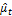. Unless the quantity to be bootstrapped is invariant to the variance of the error terms, if not, it is advisable to rescale the residuals so that they have the correct variance. The simplest type of rescaled residual is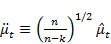(1)

The bootstrap errors here are said to be ‘resampled’ from the üt. That is, they are drawn from the empirical distribution function, or EDF, of the üt. This function assigns probability 1/n to each of the üt. Thus, each of the bootstrap error terms can take on n possible values, namely, the values of the üt, each with probability 1/n.

3.2. Parametric Bootstrap

The reason parametric bootstrap often works well is that least squares estimates and test statistics are generally not very sensitive to the distribution of the error terms. Of course, interest lies on when the distribution is assumed to be known, the parametric bootstrap DGP is;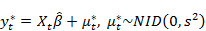(2)

Here it is assumed that the errors are normally distributed, and so the bootstrap error terms are independent normal random variates and the usual use ordinary least square estimate of the error variance. Similar methods can be used with any model estimated by maximum likelihood, but their validity generally depends on the strong assumptions inherent in maximum likelihood estimation.

3.3. Evaluation Criteria

The following statistics evaluation criteria were used to investigate and understand the bootstrap DGP methods. Also, used to investigate the impact of different proficiency level, bias, standard error, root mean square (RMSE) on SLR models and to estimate the test statistics of the functional models. To achieve this, a satisfactory degree of smoothness for the distributions including nineteen assessment conditions will be used to estimate proficiency level, bias and standard error from the two bootstrap methods, in order to determine the best model. In bias test by , , a difference of 0.1 standard deviation units is generally considered relatively large, whereas a difference of 0.25 is regarded as very large. This style will be adopted in this study. They ten assessment conditions can be classified into two categories. The first category describes the five factors and the second category describes kernel density, three test lengths and quantile- quantile plot. It is pertinent to note that 268,800 scenarios were replicated 1000 times.

These nineteen assessment conditions are described below, starting with;

The First Category - Five Factors;

a.  Factor 1:

Bootstrap method; as indicated earlier, the residual and parametric bootstrap methods were considered.

b.  Factor 2:

Restrictions; in the bootstrap methods the total of two re-scaled and seven transformations were carried out to improve the bootstrap data generating processes in the study.

c.  Factor 3:

Degree of group proficiency difference; the populations do not need to be equivalent in ability levels. Therefore, it is essential to investigate this factor since it is reflected by the magnitude of differences in the means of the examinees’ ability distributions. The bootstrapped proficiency levels, investigation and evaluation will be described in four forms; N(0, 1), N(0, σ2), N(0, s2), and N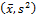denoted as (X, M, Z Q). Here, we use the standard error to get the group differences except in the last form were both were considered. Though, ,  used only the mean differences in their study. Since σ2 = 1.00001, the two forms (X, M) are approximately the same based on the simulated values, so they are treated as one form.

d.  Factor 4:

Different sample sizes 10, 14, 20, 28, 40, 50, 56, 80, 113, 160, 200, 226, 320, 452, 500, 640, 905, 1000, 3000 and 10,000 were studied. These levels represented typical small, medium, and large sample sizes.

e.  Factor 5:

Bootstrap levels (PB and NPB levels); B-Level will be 99, 199, 499, 999, 1999. These levels also represented typical small, medium, and large sample sizes and satisfies the pivotal conditions.

Next section will be the analysis and interpretation of the estimates from the iid hypothetical data set aided by an R-Statistical package.

4. Data Analysis

i.  The results obtained from the Residual bootstrap data generating process (DGP), when applied on the hypothetical data sets with fixed sample size are as follows;

4.1. Hypothetical Model (HR311), B=99, N(0, 1), n1=10000

HYPt = bo + b1A+ b2B+ e  (3)

HYPt = 23.0231205b1 + 0.043451351 b2  (4)

Standard error (0.05458350) (0.014030)   (5)

Bias (0.00652269) (0.0162333)  (6)

ii.  The results obtained from the Parametric bootstrap DGP when applied on the hypothetical data sets with fixed sample size using (3) are as follows;

4.2. Hypothetical Model (HP311), B=99, N(0, 1), n1=10000

HYPt = 23.00661205b1 + 0.039991390 b2  (7)

Standard error (0.67646) (0.0329180)   (8)

Bias (0.011196) (0.033732)    (9)

5. Interpretation of Results

This section will be based on the hypothetical bootstrap models when bootstrap DGP models with Uncorrelated Error Term from the forms X; M; Z; Q distributions.

Bias and standard error of the SLR a hypothetical data set

As the sample size increased, the bias obtained from all the residual bootstrap models (HR311), decreased at almost all estimated values, which is to be expected because of the property of estimation bias. It can also be noted that although the bias at the estimated range for parametric bootstrap models (HP311) was large (in absolute value), the estimates curves from different parametric bootstrap models were closer to one another when the sample size was 20,000 than when the sample size was 200, Table 1. Across all the conditions considered, models HR311 yielded much smaller bias and standard error than the other models at almost all score points and various conditions. The regression coefficients (b1 and b2) of HR311 and HP311 have positive relationship with HYPt and also highly significant at 5% level.

A general observation is that across different group proficiency levels, as the sample size increased, the standard error reduced; meanwhile, the differences among the different parametric bootstrap models were becoming more similar. As with the conditional bias, as the bootstrap level increased, the bias generally decreased. In Test 1, across the three different sample sizes and the three different group proficiency levels, the largest the bias and standard error were always associated with model HP311 while the smallest bias and standard error values were associated with models HR311. However, the difference in the bias between model HR311 and model HR311 in Test 2 was less than 0.0003. For Test 3, the results for including or excluding the lower end scores were not different, for HR311 still yielded the smallest bias and standard error, see Table 1 and 2. The exception was found for the group proficiency level 3- N(1, 0.25), when the sample sizes were 200, 1000 and 10000 instead of model HR311 producing the smallest the bias and standard error, model HP311 did.

Table 1. Bias of the SLR for Residual and Parametric Bootstrap Models in a Hypothetical data set.

 bootstrap Ability Sample level Level Size HR311 HP311 B=99 N (0, 1) 200 0.0842 0.1189 1000 0.0336 0.0498 10000 0.0162 0.0337 N (0, 0.9) 200 0.0365 0.0812 1000 0.0204 0.0397 10000 0.0114 0.0165 N (1, 0.25) 200 0.0748 0.1240 1000 0.0345 0.0356 10000 0.0171 0.0331 B=499 N (0, 1) 200 0.0773 0.1224 1000 0.0328 0.0598 10000 0.0160 0.0162 N (0, 0.9) 200 0.1195 0.0765 1000 0.0344 0.0347 10000 0.0159 0.0166 N (1, 0.25) 200 0.1227 0.0748 1000 0.0601 0.0345 10000 0.0171 0.0177 B=1999 N (0, 1) 200 0.1063 0.0813 1000 0.0518 0.0333 10000 0.0172 0.0297 N (0, 0.9) 200 0.1052 0.0828 1000 0.0332 0.0480 10000 0.0290 0.0170 N (1, 0.25) 200 0.1042 0.0814 1000 0.0344 0.0308 10000 0.0710 0.0289

Note. The bold is the smallest value in each row

Table 2. Standard Error of the SLR for Residual and Parametric Bootstrap Models in a Hypothetical data set.

 bootstrap Ability Sample Level Level Size HR311 HP311 B=99 N (0, 1) 200 0.0580 0.1189 1000 0.0311 0.0323 10000 0.0140 0.0329 N (0, 0.9) 200 0.0765 0.0312 1000 0.0244 0.0647 10000 0.0129 0.0334 N (1, 0.25) 200 0.0748 0.1240 1000 0.0145 0.0356 10000 0.0172 0.0331 B=499 N (0, 1) 200 0.0573 0.1224 1000 0.0328 0.0598 10000 0.0160 0.0165 N (0, 0.9) 200 0.0765 0.1216 1000 0.0344 0.0349 10000 0.0162 0.0159 N (1, 0.25) 200 0.1227 0.0748 1000 0.0345 0.0599 10000 0.0171 0.0177 B=1999 N (0, 1) 200 0.1063 0.0813 1000 0.0518 0.0333 10000 0.0273 0.0176 N (0, 0.9) 200 0.0828 0.0904 1000 0.0506 0.0332 10000 0.0170 0.0188 N (1, 0.25) 200 0.0814 0.1042 1000 0.0344 0.0308 10000 0.0710 0.0289

Note. The bold is the smallest value in each row.

6. Conclusion

The main findings are that, under all bootstrap conditions, the HR311 functional models produced smaller bias and standard error than HP311 functional models. The regression coefficients (b1 and b2) of HR311 and HP311 have positive relationship with HYPt and are also highly significant at 5% level. The result shows a significant difference between the performances of the bootstrap methods used, namely; residual and parametric bootstrap techniques. From the analysis, the largest bias and standard error were always associated with model HP311 while the smallest bias and standard error values were associated with models HR311. The exception was found for the group proficiency level 3- N(1, 0.25), when the sample sizes were 200, 1000 and 10000 instead of model HR311 producing the smallest bias and standard error, model HP311 did. The significantly better performance of the residual bootstrap indicates the possible use of this technique in assessment of comparative performance and the capability of yielding very accurate, efficient and sufficient statistical inference under the several assessment conditions considered.

References

1. Efron, B. (1983). Estimating the error rate of a prediction rule: improvements on cross-validation. J. Am. Statist. Assoc. 78, 316-331.
2. Politis, D. N. and Romano J. P. (1994a). The stationary bootstrap. J. Am. Statist. Assoc. 89, 1303-1313.
3. Paparoditis, E., and Politis, D. N. (2001a). Tapered block bootstrap.Biometrika88, 1105-1119.
4. Lahiri, S. N. (2006). Bootstrap Methods: A Review. In Frontiers in Statistics (J. Fan and H. L. Koul, editors) 231-265, Imperial College Press, London.
5. Paparoditis, E., and Politis, D. N. (2005). Bootstrapping unit root tests for autoregressive time series.J. Am. Statist. Assoc.100, 545-553.
6. Schimek, M. G. (2000) Smoothing and Regression. Wiley, Hoboken.
7. Chernick, M. R. (1999). Bootstrap Methods: A Practitioners Guide. Wiley, New York.
8. Chernick, M. R. and LaBudde, R. (2011). An Introduction to the Bootstrap with Applications in R Wiley, Hoboken.
9. Paparoditis, E., and Politis, D. N. (2001b). A Markovian local resampling scheme for nonparametric estimators in time series analysis.Econ. Theory1, 540-566.
10. Paparoditis, E. (2005). Testing the fit of a vector autoregressive moving average model.J. Time Series Anal.26, 543-568.
11. Politis, D. N., and White, H. (2004). Automatic block-length selection for the dependent bootstrap.Econ. Rev.23, 53-70.
12. Politis, K. (2003). Semiparametric estimation for non-ruin probabilities.Scand. Act. J., 75-96.
13. Wilcox, R., and Keselman, H. (2006). Detecting heteroscedasticity in a simple regression model via quantile regression slopes.J. Statist. Comput. Simul.76, 705-712.
14. William G. J. and David A. A. (2014), Bootstrap Confidence Regions for Multidimensional Scaling Solutions. American Journal of Political Science, 58 (1); 264-278.
15. Acha, C. K. (2014a) Parametric Bootstrap Methods for Parameter Estimation in SLR Models. International Journal of Econometrics and Financial Management, 2 (5), 175-179. Doi: 10.12691/ijefm-2-5-2.
16. Acha, C. K. (2014b) Bootstrapping Normal and Binomial Distributions. International Journal of Econometrics and Financial Management, 2 (6), 253– 256. Doi: 10.12691/ijefm-2-6-2.
17. Acha, C. K. and Acha I. A. (2015) Smooth Bootstrap Methods on External Sector Statistics. International Journal of Econometrics and Financial Management, 3 (3), 115–120. Doi: 10.12691/ijefm-3-3-2.
18. Wang, T., Lee, W., Brennan, R. L. & Kolen, M. J. (2008). A comparison of frequency estimation and chained equipercentile methods under the common-item nonequivalent groups design. Applied Psychological Measurement, 32, 632-651.
19. Wang, T. & Brennan, R. L. (2009). A modified frequency estimation equating method for the common-item nonequivalent groups design estimating random error in equipercentile equating. Applied Psychological Measurement, 33, 118-132.
20. Hanson, B. A., & B´eguin, A. A. (2002). Obtaining a common scale for item response theory item parameters using separate versus concurrent estimation in the common-item equating design. Applied Psychological Measurement, 26, 3-24.
21. Lee, W. C., & Ban, J. C. (2007). Comparison of Three IRT Linking Procedures in the Random Groups Equating Design. Center for Advanced Studies in Measurement and Assessment CASMA Research Report (23)1-27.

 Contents 1. 2. 3. 3.1. 3.2. 3.3. 4. 4.1. 4.2. 5. 6.
Article ToolsAbstractPDF(203K)